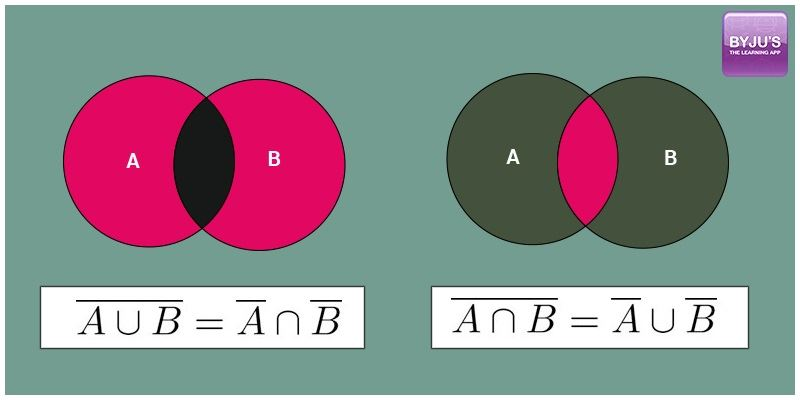# De Morgan's Law and Properties of Complement of a Set

De Morgan’s law states that ‘The complement of the union of two sets A and B is equal to the intersection of the complement of the sets A’ and B’. Also, according to De Morgan’s law, the complement of the intersection of two sets A and B is equal to the union of the complement of the sets A and B i.e.,

(A∪B)’ = A’ ∩ B’And (A ∩ B)’ = A’ ∪ B’

## What is the complement of a Set?

The complement of a set is defined as

A’ = {x : x ∈ U and x ∉ A}

where A’ denotes the complement.

## Complement of Sets Properties

i) Complement Laws: The union of a set A and its complement A’ gives the universal set U of which, A and A’ are a subset.

A ∪ A’ = U

Also, the intersection of a set A and its complement A’ gives the empty set ∅.

A ∩ A’ = ∅

For Example: If U = {1 , 2 , 3 , 4 , 5 } and A = {1 , 2 , 3 } then A’ = {4 , 5}. From this it can be seen that

A ∪ A’ = U = { 1 , 2 , 3 , 4 , 5}

Also

A ∩ A’ = ∅

ii) Law of Double Complementation: According to this law if we take the complement of the complemented set A’ then, we get the set A itself.

(A’)’ = A

In the previous example we can see that, if U = {1 , 2 , 3 , 4 , 5} and A = {1 , 2 ,3} then A’ ={4 , 5}. Now if we take the complement of set ‘A’ we get,

(A’)’ = {1 , 2 , 3} = A

This gives back the set A itself.

iii) Law of empty set and universal set:

According to this law the complement of the universal set gives us the empty set and vice-versa i.e.,

∅’ = U And U’ = ∅

This law is self-explanatory.

To understand this law better let us consider the following example:

### Solved Problem

Example:

A universal set U which consists of all the natural numbers which are multiples of 3, less than or equal to 20. Let A be a subset of U which consists of all the even numbers and the set B is also a subset of U consisting of all the prime numbers. Verify De Morgan Law.

Solution: We have to verify (A ∪ B)’ = A’ ∩ B’ and (A ∩ B)’ = A’∪B’. Given that,

U = {3 , 6 , 9 , 12 , 15 , 18}

A = {6 , 12 , 18}

B = {3}

The union of both A and B can be given as,

A ∪ B = {3 , 6 , 12 , 18}

The complement of this union is given by,

(A ∪ B)’={9 , 15}

Also, the intersection and its complement are given by:

A ∩ B = ∅

(A ∩ B)’ = {3 , 6 , 9 , 12 , 15 ,18}

Now, the complement of the sets A and B can be given as:

A’ = {3 , 9 , 15}

B’ = {6 , 9 , 12 , 15 , 18}

Taking the union of both these sets, we get,

A’∪B’ = {3 , 6 , 9 , 12 , 15 ,18}

And the intersection of the complemented sets is given as,

A’ ∩ B’ = {9 , 15}

We can see that:

(A ∪ B)’ = A’ ∩ B’ = {9 , 15}

And also,

(A ∩ B)’ = A’ ∪ B’ = {3 , 6 , 9 , 12 , 15 ,18}

Hence, the above result is true in general and is known as De Morgan Law.

## Video Lesson on What are SetsThe world of sets has a lot more to share with you. To dig deeper please visit our sit BYJU’S and explore the world of innumerable possibilities.

Test your knowledge on Properties Of Complement Of Set